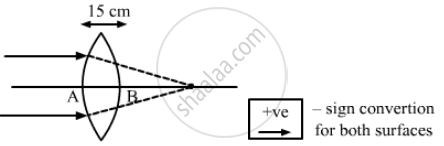Department of Pre-University Education, KarnatakaPUC Karnataka Science Class 12
Advertisement Remove all ads

# A Biconvex Thick Lens is Constructed with Glass (μ = 1.50). Each of the Surfaces Has a Radius of 10 Cm and the Thickness at the Middle is 5 Cm. - Physics

Sum

A biconvex thick lens is constructed with glass (μ = 1.50). Each of the surfaces has a radius of 10 cm and the thickness at the middle is 5 cm. Locate the image of an object placed far away from the lens.

Advertisement Remove all ads

#### Solution

Given,
Biconvex lens with each surface has a radius (R1 R2 = R) = 10 cm,
and thickness of the lens (t) = 5 cm
Refractive index of the lens (μ) = 1.50
Object is at infinity (∴ u = ∞ )First refraction takes place at A.
We know that,

$\frac{\mu_g}{v} - \frac{\mu_a}{u} = \frac{\mu_g - \mu_a}{R}$

$\Rightarrow \frac{1 . 5}{v} - \left( - \frac{1}{\infty} \right) = \frac{1 . 5 - 1}{10}$

$\Rightarrow \frac{1 . 5}{v} = \frac{0 . 5}{10}$

$\Rightarrow v = 30 \text{ cm }$
Now, the second refraction is at B.
For this, a virtual object is the image of the previous refraction.
Thus, u = (30 − 5) = 25 cm

$\frac{\mu_a}{v} - \frac{\mu_g}{u} = \frac{\mu_a - \mu_g}{R}$

$\Rightarrow \frac{1}{v} - \frac{1 . 5}{25} = \frac{1 - 1 . 5}{10}$

$\Rightarrow \frac{1}{v} - \frac{1 . 5}{25} = \frac{- 0 . 5}{10}$
$\Rightarrow v = 9 . 1 cm$
Hence, the image is formed 9.1 cm far from the second refraction or second surface of the lens.

Is there an error in this question or solution?
Advertisement Remove all ads

#### APPEARS IN

HC Verma Class 11, 12 Concepts of Physics 1
Chapter 18 Geometrical Optics
Q 41 | Page 415
Advertisement Remove all ads
Advertisement Remove all ads
Share
Notifications

View all notifications

Forgot password?